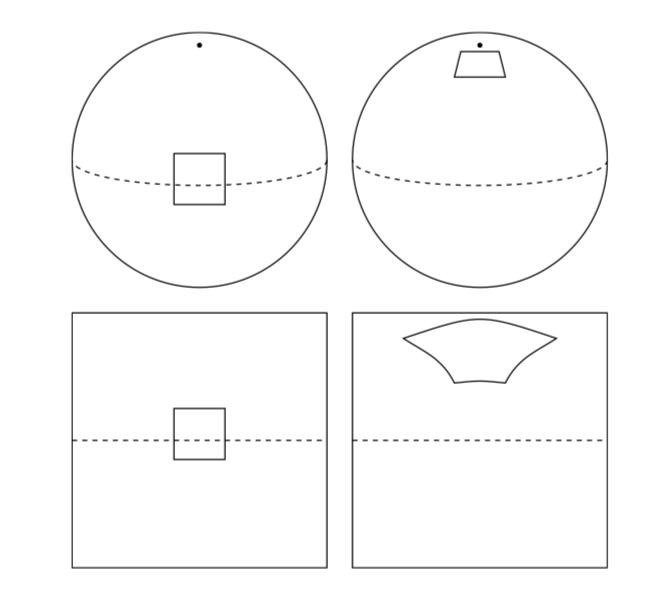# Eyes on the Sphere

Source: Deep Learning on Medium## An Overview of Spherical CNNs, Best Paper Award in the 2018 ICLR Conference by Taco Cohen and his team!

Convolutional Neural Networks (CNNs), which is a class of deep learning neural networks, have become the go-to method for 2D image detection/classification as it produce accurate results without taking too much computing power or time. You can find out more about how it works here and the original motivation, which was inspired by human brain activity here. However, with the increased popularity of self driving cars, omnidirectional images, and other 3D maps (such as wind maps, drones, temperature maps, etc.) we arrive at the challenge of conducting image recognition on spherical images.

Note that we are unable to just project the image on the sphere onto a plane because the regions would be immensely distorted.

In this figure, we see that a region highlighted in the middle (aligned over the equator) would produce what looks like a square when projected onto a plane. That same region on the same sphere where the sphere is oriented differently (rotated some number of degrees) and then projected again onto the plane now looks nothing like the sphere. Nothing is preserved, so it is very difficult for a traditional 2D CNN to detect regions on a spherical image.

Similarly, if I asked you what Antarctica looked like, your views are probably heavily influenced by what sort of map you are familiar with. Consider the following views below which I have collected from a variety of images I found on Google.

Antarctica looks very different in all of these pictures, which are just rotated views of the globe. So how do we construct a CNN to detect images on the sphere? In Cohen’s paper on Spherical CNNs, they present a Fast Fourier Transform based algorithm to detect images on a sphere that solves two primary challenges. First, there is no symmetrical grid that we can fit to a sphere the way we can a plane. This means that we can’t just consider a single pixel on the sphere. Second, if we consider the set of all table of all possible rotations of the sphere, then there is something on the order of O(n⁶) entries in this table, which leads to computational inefficiency. In this overview, we will go over more of the theory behind spherical CNNs and why it works and present some results.

In 2D CNNs (as shown above), we detect some sequence of pixels, with the invariant being translations. So it shouldn’t matter where the bird is located on the image. In other words, we can slide the bird around anywhere on the image and we would still be able to detect that the image is an image of a bird.

In spherical images, the invariant we want to preserve are rotations. To do this, we define the following terms that will be useful to understand the theoretical foundations of spherical CNNs.

Unit Sphere
The unit sphere is just some sphere with radius 1 and we will be considering images defined on it.

Spherical Signals
These are functions defined on the sphere f : S² -> R³ where is the number of channels (in this example 3, consider R, G, B channels)

Rotation Group on the Sphere SO(3)
The Rotation Group on the sphere can be thought of as the group of all possible rotations on the sphere (around the origin in 3D space). Since rotations are linear transforms in R³, we can treat them like matrices. Furthermore, we verify that SO(3) is a group through the following: composing two rotations results in another rotation, every rotation has a unique inverse rotation, and the identity map satisfies the definition of a rotation, the set of all rotations is a group under composition. But because rotations are not commutative, it is a nonabelian group. Furthermore, the rotation group has a natural structure that makes it a manifold for which group operations are smoothly differentiable, which makes it a 3 dimmensional and compact. It is also a Lie group. A bit of a tangent: it is not extremely intuitive as to how a group can both be a manifold and an algebraic structure or why it is useful, but we can consider SO(2), which is the group of rotations on the 2D plane. Geometrically, it looks like a circle (so it is also a manifold). Note that the rotation group preserves distance (so it’s an isometry) and orientation. This will be important when we prove invariants later on.

In the image above, note that x denotes the original image and phi(x) denotes some filter (or a function) applied to the image. We now want to verify that for every rotation R, R(phi(x)) will give us the same thing as phi(R(x)). We define the following notation to help us do this

We define the above rotation operator LR that takes in a function f and then creates the rotated function. Note that this is the same as computing the function on the rotated image.

Now we will show that the inner product between a spherical signal (image) and filter (function) is invariant. This will help us prove that spherical correlations are held invariant for rotations, which is the bulk of the proof for the theory of spherical CNNs. First note that the volume of some spherical height map does not change when rotated, which gives us the following identity.

This means that the rotation operator is a unitary operator, which preserves dot product, is normal, and preserves orientation. It also implies that the inverse of the rotation Operator is adjoint to the rotation operator, which means that if A, B are two adjoint operators, (Ax, y) = (x, By). See proof below.